Open in App
Not now

# Implement Deep Autoencoder in PyTorch for Image Reconstruction

• Last Updated : 13 Jul, 2021

Since the availability of staggering amounts of data on the internet, researchers and scientists from industry and academia keep trying to develop more efficient and reliable data transfer modes than the current state-of-the-art methods. Autoencoders are one of the key elements found in recent times used for such a task with their simple and intuitive architecture.

Broadly, once an autoencoder is trained, the encoder weights can be sent to the transmitter side and the decoder weights to the receiver side. This way, the transmitter side can send data in an encoded format(thus saving them time and money) while the receiver side can receive the data at much less overhaul. This article will explore an interesting application of autoencoder, which can be used for image reconstruction on the famous MNIST digits dataset using the Pytorch framework in Python.

## Autoencoders

As shown in the figure below, a very basic autoencoder consists of two main parts:

1. An Encoder and,
2. A Decoder

Through a series of layers, the encoder takes the input and takes the higher dimensional data to the latent low dimension representation of the same values. The decoder takes this latent representation and outputs the reconstructed data.

For a deeper understanding of the theory, the reader is encouraged to go through the following article: ML | Auto-Encoders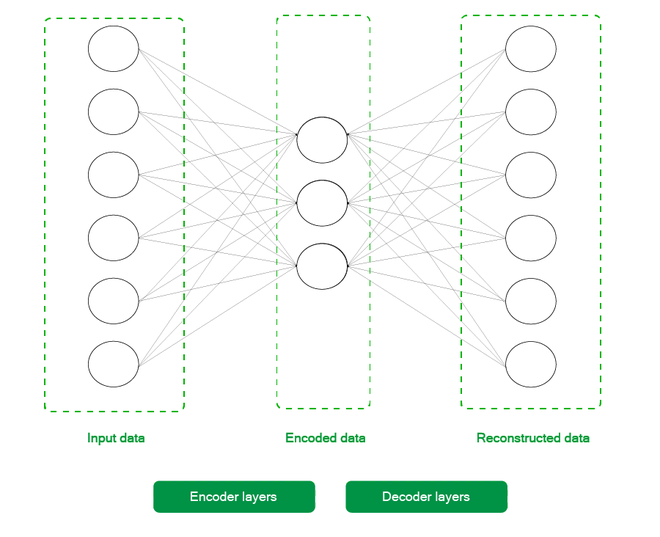A basic 2 layer Autoencoder

## Installation:

Aside from the usual libraries like Numpy and Matplotlib, we only need the torch and torchvision libraries from the Pytorch toolchain for this article. You can use the following command to get all these libraries.

pip3 install torch torchvision torchaudio numpy matplotlib

Now onto the most interesting part, the code. The article assumes a basic familiarity with the PyTorch workflow and its various utilities, like Dataloaders, Datasets and Tensor transforms. For a quick refresher of these concepts, the reader is encouraged to go through the following articles:

The code is divided into 5 different steps for a better flow of the material and is to be executed sequentially for proper work. Each step also has some points at its start, which can help the reader better understand that step’s code.

## Stepwise implementation:

Step 1: Loading data and printing some sample images from the training set.

• Initializing Transform: Firstly, we initialize the transform which would be applied to each entry in the attained dataset. Since Tensors are internal to Pytorch’s functioning, we first convert each item to a tensor and normalize them to limit the pixel values between 0 & 1. This is done to make the optimization process easier and faster.
• Downloading Dataset: Then, we download the dataset using the torchvision.datasets utility and store it on our local machine in the folder ./MNIST/train and ./MNIST/test for both training and testing sets. We also convert these datasets into data loaders with batch sizes equal to 256 for faster learning. The reader is encouraged to play around with these values and expect consistent results.
• Plotting Dataset: Lastly, we randomly print out 25 images from the dataset to better view the data we’re dealing with.

Code:

## Python

 `# Importing the necessary libraries``import` `numpy as np``import` `matplotlib.pyplot as plt``import` `torchvision``import` `torch``plt.rcParams[``'figure.figsize'``] ``=` `15``, ``10`` ` `# Initializing the transform for the dataset``transform ``=` `torchvision.transforms.Compose([``    ``torchvision.transforms.ToTensor(),``    ``torchvision.transforms.Normalize((``0.5``), (``0.5``))``])`` ` `# Downloading the MNIST dataset``train_dataset ``=` `torchvision.datasets.MNIST(``    ``root``=``"./MNIST/train"``, train``=``True``,``    ``transform``=``torchvision.transforms.ToTensor(),``    ``download``=``True``)`` ` `test_dataset ``=` `torchvision.datasets.MNIST(``    ``root``=``"./MNIST/test"``, train``=``False``,``    ``transform``=``torchvision.transforms.ToTensor(),``    ``download``=``True``)`` ` `# Creating Dataloaders from the``# training and testing dataset``train_loader ``=` `torch.utils.data.DataLoader(``    ``train_dataset, batch_size``=``256``)``test_loader ``=` `torch.utils.data.DataLoader(``    ``test_dataset, batch_size``=``256``)`` ` `# Printing 25 random images from the training dataset``random_samples ``=` `np.random.randint(``    ``1``, ``len``(train_dataset), (``25``))`` ` `for` `idx ``in` `range``(random_samples.shape[``0``]):``    ``plt.subplot(``5``, ``5``, idx ``+` `1``)``    ``plt.imshow(train_dataset[idx][``0``][``0``].numpy(), cmap``=``'gray'``)``    ``plt.title(train_dataset[idx][``1``])``    ``plt.axis(``'off'``)`` ` `plt.tight_layout()``plt.show()`

Output: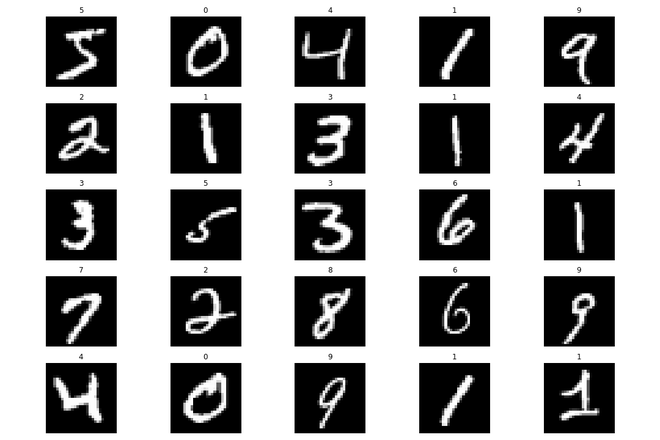Random samples from the training set

Step 2: Initializing the Deep Autoencoder model and other hyperparameters

In this step, we initialize our DeepAutoencoder class, a child class of the torch.nn.Module. This abstracts away a lot of boilerplate code for us, and now we can focus on building our model architecture which is as follows: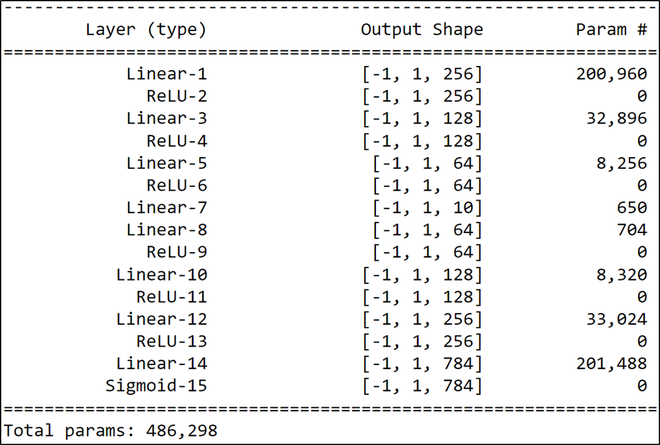Model Architecture

As described above, the encoder layers form the first half of the network, i.e., from Linear-1 to Linear-7, and the decoder forms the other half from Linear-10 to Sigmoid-15. We’ve used the torch.nn.Sequential utility for separating the encoder and decoder from one another. This was done to give a better understanding of the model’s architecture. After that, we initialize some model hyperparameters such that the training is done for 100 epochs using the Mean Square Error loss and Adam optimizer for the learning process.

## Python

 `# Creating a DeepAutoencoder class``class` `DeepAutoencoder(torch.nn.Module):``    ``def` `__init__(``self``):``        ``super``().__init__()        ``        ``self``.encoder ``=` `torch.nn.Sequential(``            ``torch.nn.Linear(``28` `*` `28``, ``256``),``            ``torch.nn.ReLU(),``            ``torch.nn.Linear(``256``, ``128``),``            ``torch.nn.ReLU(),``            ``torch.nn.Linear(``128``, ``64``),``            ``torch.nn.ReLU(),``            ``torch.nn.Linear(``64``, ``10``)``        ``)``         ` `        ``self``.decoder ``=` `torch.nn.Sequential(``            ``torch.nn.Linear(``10``, ``64``),``            ``torch.nn.ReLU(),``            ``torch.nn.Linear(``64``, ``128``),``            ``torch.nn.ReLU(),``            ``torch.nn.Linear(``128``, ``256``),``            ``torch.nn.ReLU(),``            ``torch.nn.Linear(``256``, ``28` `*` `28``),``            ``torch.nn.Sigmoid()``        ``)`` ` `    ``def` `forward(``self``, x):``        ``encoded ``=` `self``.encoder(x)``        ``decoded ``=` `self``.decoder(encoded)``        ``return` `decoded`` ` `# Instantiating the model and hyperparameters``model ``=` `DeepAutoencoder()``criterion ``=` `torch.nn.MSELoss()``num_epochs ``=` `100``optimizer ``=` `torch.optim.Adam(model.parameters(), lr``=``1e``-``3``)`

Step 3: Training loop

The training loop iterates for the 100 epochs and does the following things:

• Iterates over each batch and calculates loss between the outputted image and the original image(which is the output).
• Averages out the loss for each batch and stores images and their outputs for each epoch.

After the loop ends, we plot out the training loss to better understand the training process. As we can see, that the loss decreases for each consecutive epoch, and thus the training can be deemed successful.

## Python

 `# List that will store the training loss``train_loss ``=` `[]`` ` `# Dictionary that will store the``# different images and outputs for ``# various epochs``outputs ``=` `{}`` ` `batch_size ``=` `len``(train_loader)`` ` `# Training loop starts``for` `epoch ``in` `range``(num_epochs):``       ` `    ``# Initializing variable for storing ``    ``# loss``    ``running_loss ``=` `0``     ` `    ``# Iterating over the training dataset``    ``for` `batch ``in` `train_loader:``           ` `        ``# Loading image(s) and``        ``# reshaping it into a 1-d vector``        ``img, _ ``=` `batch  ``        ``img ``=` `img.reshape(``-``1``, ``28``*``28``)``         ` `        ``# Generating output``        ``out ``=` `model(img)``         ` `        ``# Calculating loss``        ``loss ``=` `criterion(out, img)``         ` `        ``# Updating weights according``        ``# to the calculated loss``        ``optimizer.zero_grad()``        ``loss.backward()``        ``optimizer.step()``         ` `        ``# Incrementing loss``        ``running_loss ``+``=` `loss.item()``     ` `    ``# Averaging out loss over entire batch``    ``running_loss ``/``=` `batch_size``    ``train_loss.append(running_loss)``     ` `    ``# Storing useful images and``    ``# reconstructed outputs for the last batch``    ``outputs[epoch``+``1``] ``=` `{``'img'``: img, ``'out'``: out}`` ` ` ` `# Plotting the training loss``plt.plot(``range``(``1``,num_epochs``+``1``),train_loss)``plt.xlabel(``"Number of epochs"``)``plt.ylabel(``"Training Loss"``)``plt.show()`

Output: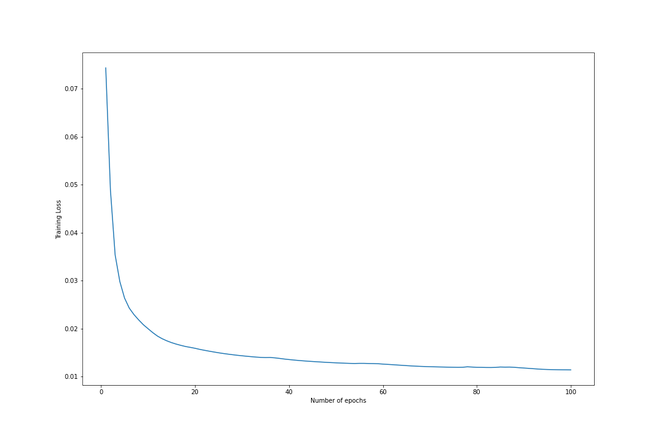Training loss vs. Epochs

Step 4: Visualizing the reconstruction

The best part of this project is that the reader can visualize the reconstruction of each epoch and understand the iterative learning of the model.

• We firstly plot out the first 5 reconstructed(or outputted images) for epochs = [1, 5, 10, 50, 100].
• Then we also plot the corresponding original images on the bottom for comparison.

We can see how the reconstruction improves for each epoch and gets very close to the original by the last epoch.

## Python

 `# Plotting is done on a 7x5 subplot``# Plotting the reconstructed images`` ` `# Initializing subplot counter``counter ``=` `1`` ` `# Plotting reconstructions``# for epochs = [1, 5, 10, 50, 100]``epochs_list ``=` `[``1``, ``5``, ``10``, ``50``, ``100``]`` ` `# Iterating over specified epochs``for` `val ``in` `epochs_list:``   ` `      ``# Extracting recorded information``    ``temp ``=` `outputs[val][``'out'``].detach().numpy()``    ``title_text ``=` `f``"Epoch = {val}"``     ` `    ``# Plotting first five images of the last batch``    ``for` `idx ``in` `range``(``5``):``        ``plt.subplot(``7``, ``5``, counter)``        ``plt.title(title_text)``        ``plt.imshow(temp[idx].reshape(``28``,``28``), cmap``=` `'gray'``)``        ``plt.axis(``'off'``)``         ` `        ``# Incrementing the subplot counter``        ``counter``+``=``1`` ` `# Plotting original images`` ` `# Iterating over first five``# images of the last batch``for` `idx ``in` `range``(``5``):``     ` `    ``# Obtaining image from the dictionary``    ``val ``=` `outputs[``10``][``'img'``]``     ` `    ``# Plotting image``    ``plt.subplot(``7``,``5``,counter)``    ``plt.imshow(val[idx].reshape(``28``, ``28``),``               ``cmap ``=` `'gray'``)``    ``plt.title(``"Original Image"``)``    ``plt.axis(``'off'``)``     ` `    ``# Incrementing subplot counter``    ``counter``+``=``1`` ` `plt.tight_layout()``plt.show()`

Output: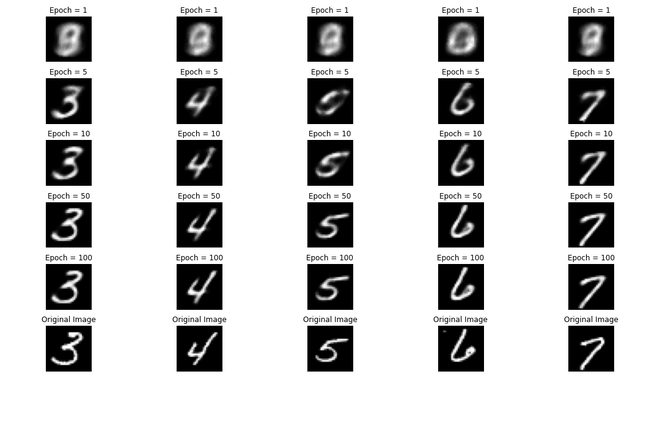Visualizing the reconstruction from the data collected during the training process

Step 5: Checking performance on the test set.

Good practice in machine learning is to check the model’s performance on the test set also. To do that, we do the following steps:

• Generate outputs for the last batch of the test set.
• Plot the first 10 outputs and corresponding original images for comparison.

As we can see, the reconstruction was excellent on this test set also, which completes the pipeline.

## Python

 `# Dictionary that will store the different``# images and outputs for various epochs``outputs ``=` `{}`` ` `# Extracting the last batch from the test ``# dataset``img, _ ``=` `list``(test_loader)[``-``1``]`` ` `# Reshaping into 1d vector``img ``=` `img.reshape(``-``1``, ``28` `*` `28``)`` ` `# Generating output for the obtained``# batch``out ``=` `model(img)`` ` `# Storing information in dictionary``outputs[``'img'``] ``=` `img``outputs[``'out'``] ``=` `out`` ` `# Plotting reconstructed images``# Initializing subplot counter``counter ``=` `1``val ``=` `outputs[``'out'``].detach().numpy()`` ` `# Plotting first 10 images of the batch``for` `idx ``in` `range``(``10``):``    ``plt.subplot(``2``, ``10``, counter)``    ``plt.title(``"Reconstructed \n image"``)``    ``plt.imshow(val[idx].reshape(``28``, ``28``), cmap``=``'gray'``)``    ``plt.axis(``'off'``)`` ` `    ``# Incrementing subplot counter``    ``counter ``+``=` `1`` ` `# Plotting original images`` ` `# Plotting first 10 images``for` `idx ``in` `range``(``10``):``    ``val ``=` `outputs[``'img'``]``    ``plt.subplot(``2``, ``10``, counter)``    ``plt.imshow(val[idx].reshape(``28``, ``28``), cmap``=``'gray'``)``    ``plt.title(``"Original Image"``)``    ``plt.axis(``'off'``)`` ` `    ``# Incrementing subplot counter``    ``counter ``+``=` `1`` ` `plt.tight_layout()``plt.show()`

Output: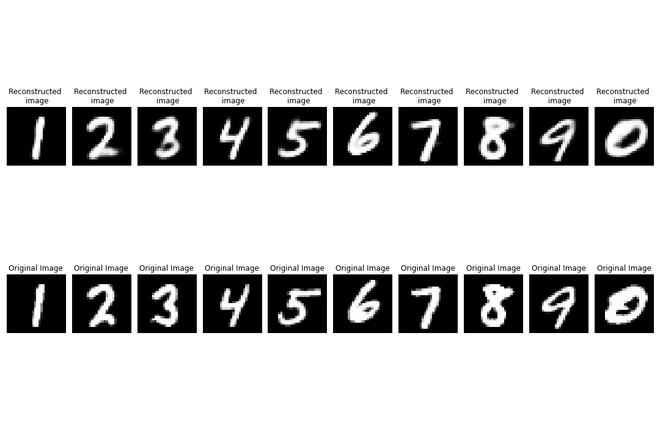Verifying performance on the test set

## Conclusion:

Autoencoders are fast becoming one of the most exciting areas of research in machine learning. This article covered the Pytorch implementation of a deep autoencoder for image reconstruction. The reader is encouraged to play around with the network architecture and hyperparameters to improve the reconstruction quality and the loss values.

My Personal Notes arrow_drop_up